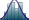×
Get Full Access to albany - Class Notes - Week 1
Get Full Access to albany - Class Notes - Week 1

×

ALBANY / OTHER / APSY 101 / What procedure is used in descriptive statistics?

# What procedure is used in descriptive statistics? Description

##### Description: These notes cover ALL of the mini-chapter of chapter 2
4 Pages 81 Views 3 Unlocks
Reviews

STATISTICAL SUPPLEMENT

## What procedure is used in descriptive statistics?1. Psychologists use statistics to make sense of the data they  collect

1. ANALYZING THE DATA

1. Statistical analysis allows researchers to  discover if their predictions were correct

∙ Raw Data = Actual Scores

2. Descriptive Statistics – uses mathematical  procedures in an objective, uniform way to  describe different aspects of numerical data  (ex. Computing GPA)

3. Inferential Statistics – uses probability theory  to make sound decisions about which results  might have occurred simply through chance  variation

A.Descriptive Statistics

i. Descriptive Statistics – provides a summary picture of  patterns in the data

## How is histogram different from a bar graph?Don't forget about the age old question of What are the four types of biological molecules?
Don't forget about the age old question of What is the galactic plane?

ii. Describes sets of scores collected from one experimental  participant (or different groups)

iii. Describes relationships among variables

1. Frequency Distributions

a. Frequency Distribution – a summary of how frequently  each of the various scores occurs

b. Step 1  rank-order scores from highest to lowest Step  2  group rank-ordered scores into a smaller number of If you want to learn more check out What is the inferior part of the thorax?

categories (intervals) Step 3  construct a table listing  the intervals from highest to lowest

2. Graphs

a. The simplest type of graph is a bar graph

b. Histogram – similar to a bar graph except with number  categories instead of name categories

## What pertains to the simplest measure of variability?If you want to learn more check out What is the modern development in microbiology?
We also discuss several other topics like What are the four d’s to describe abnormality?

3. Measures of Central Tendency

a. Measure of Central Tendency – a single representative  score that can be used as an index of the most typical  score obtained by a group of participants

b. Three Different Measures of Central Tendency  MODE,  MEDIAN, MEAN

c. Mode – The score that occurs more often than any other ∙ Easiest index of central tendency to determine, but  least useful

d. Median – a central score (separates upper and lower  half of scores)

∙ ODD # OF SCORES = middle number

∙ EVEN # OF SCORES = average of middle numbers

e. Mean – average of ALL scores. Add up all scores and  divide by total number of scores

∙ M = ((ΣX)

N )

∙ M: Mean, X: Individual Score, Σ : Summation of  What Immediately Follows, N: Total Number of

Scores

f. Unlike the median, the mean IS affected by the specific  values of all scores in the distribution

4. Variability

a. Measures of Variability – statistic indicating how tightly  the scores in a set of observations cluster together  b. Range – simplest measure of variability; difference  between the highest and lowest score  We also discuss several other topics like Why might scholars not be sure that homer existed?

c. Standard Deviation (SD) – a measure of variability that  indicates the average difference between the scores  d. You need to know the mean to figure out standard  deviation (individual scores also need to be known)

2

∙ SD = √Σ( X− M)2

N

∙ (X-M) = individual score minus mean AKA

deviation score

∙ The mean is subtracted from each score, and

each resulting score is squared (to eliminate

negative values)

e. Mean of deviations calculated by summing them up ( Σ ) and dividing by the number of observations (N) [ √  take square root]

f. The larger the standard deviation, the more spread out  the scores are

5. Correlation

a. Correlation Coefficient (r) – measure of the nature and  strength of the relationship between two variables  b. The further a coefficient is from 0 (in either direction)  the more closely related the two variables are [higher  coefficients permit better predictions of one variable] B.Inferential Statistics

i. Inferential Statistics tells us which inferences we can  make from our samples and which conclusions we can  legitimately draw from our data

1. The Normal Curve

a. Normal Curve – symmetrical curve that represents the  distribution of scores [bell shaped]

b. The median, mode, AND mean values are the same 2. Statistical Significance

a. Significant Difference – difference between

experimental groups/conditions occurring by chance  with a probability of less than 5 out of 100 (p < .05) b. T-Test – analysis of two populations means through the  use of statistical examination; combining information  about means and standard deviations

3

2. BECOMING A WISE CONSUMER OF  STATISTICS

1. Large samples are less likely to be misleading than small ones

4

Page ExpiredIt looks like your free minutes have expired! Lucky for you we have all the content you need, just sign up here